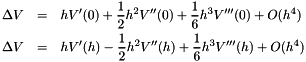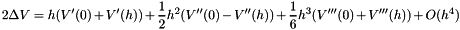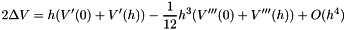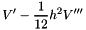Gromacs  2019-beta2

#include <gromacs/tables/quadraticsplinetable.h>

## Description

This class interpolates a function specified either as an analytical expression or from user-provided table data.

At initialization, you provide the reference function of vectors as a list of tuples that contain a brief name, the function, and derivative for each function to tabulate. To create a table with two functions this initializer list can for instance look like

{ {"LJ6", lj6Func, lj6Der}, {"LJ12", lj12Func, lj12Der} }


The names are only used so exceptions during initialization can be traced to a specific table.

When interpolating, there are methods to interpolate either 1, 2, or 3 functions in one go. By default these interpolation routines will operate on tables with the same number of functions as specified in the interpolation method (debug builds check that this is consistent with the table). However, it is also possible to use optional template parameters that specify the total number of functions in a table, and what function index to interpolate. For instance, to interpolate the derivative of the second function (i.e., index 1) in a multi-function-table with three functions in total, you can write

table.evaluateDerivative<3,1>(x,&der);


Here too, debug builds will check that the template parameters are consistent with the table.

The table data is internally adjusted to guarantee that the interpolated derivative is the true derivative of the interpolated potential, which is important to avoid systematic errors for the common case when the derivative is concave/convex in the entire interval. We do this by expressing the difference in the function value at a small offset h relative to a reference value in position 0 with a forward Taylor series expanded around 0, and then doing the opposite of expressing difference in the function at position 0 relative to a reference value in position h when using a backward Taylor expansion:To make the second term symmetric too, we can replace it with the average of the Taylor expansion at 0 and h (i.e., using the third derivative). This givesThus, if we replace the derivative in the internal quadratic table data withwe will cancel the h^3 term in the error. This will make the integral of the forces match the potential much better (The h^4 term actually disappears, so when summing over 1/h points the remaining error will be O(h^4).

While it is possible to create tables only from function values (i.e., no derivatives), it is recommended to provide derivatives for higher accuracy and to avoid issues with numerical differentiation. Note that the table input should be smooth, i.e. it should not contain noise e.g. from an (iterative) Boltzmann inversion procedure - you have been warned.

Note
This class is responsible for fundamental interpolation of any function, which might or might not correspond to a potential. For this reason both input and output derivatives are proper function derivatives, and we do not swap any signs to get forces directly from the table.
There will be a small additional accuracy loss from the internal operation where we calculate the epsilon offset from the nearest table point, since the integer part we subtract can get large in those cases. The absolute such error both in the function and derivative value will be roughly f''*x*GMX_REAL_EPS, where x is the argument and f'' the second derivative. While this is technically possible to solve with extended precision arithmetics, that would introduce extra instructions in some highly performance-sensitive code parts. For typical GROMACS interaction functions the derivatives will decay faster than the potential, which means it will never play any role. For other functions it will only cause a small increase in the relative error for arguments where the magnitude of the function or derivative is very small. Since we typically sum several results in GROMACS, this should never show up in any real cases, and for this reason we choose not to do the extended precision arithmetics.
These routines are not suitable for table ranges starting far away from zero, since we allocate memory and calculate indices starting from range zero for efficiency reasons.

## Public Member Functions

QuadraticSplineTable (std::initializer_list< AnalyticalSplineTableInput > analyticalInputList, const std::pair< real, real > &range, real tolerance=defaultTolerance)
Initialize table data from function. More...

QuadraticSplineTable (std::initializer_list< NumericalSplineTableInput > numericalInputList, const std::pair< real, real > &range, real tolerance=defaultTolerance)
Initialize table data from tabulated values and derivatives. More...

template<int numFuncInTable = 1, int funcIndex = 0, typename T >
void evaluateFunctionAndDerivative (T r, T *functionValue, T *derivativeValue) const
Evaluate both function and derivative, single table function. More...

template<int numFuncInTable = 1, int funcIndex = 0, typename T >
void evaluateFunction (T r, T *functionValue) const
Evaluate function value only, single table function. More...

template<int numFuncInTable = 1, int funcIndex = 0, typename T >
void evaluateDerivative (T r, T *derivativeValue) const
Evaluate function derivative only, single table function. More...

template<int numFuncInTable = 2, int funcIndex0 = 0, int funcIndex1 = 1, typename T >
void evaluateFunctionAndDerivative (T r, T *functionValue1, T *derivativeValue1, T *functionValue2, T *derivativeValue2) const
Evaluate both function and derivative, two table functions. More...

template<int numFuncInTable = 2, int funcIndex0 = 0, int funcIndex1 = 1, typename T >
void evaluateFunction (T r, T *functionValue1, T *functionValue2) const
Evaluate function value only, two table functions. More...

template<int numFuncInTable = 2, int funcIndex0 = 0, int funcIndex1 = 1, typename T >
void evaluateDerivative (T r, T *derivativeValue1, T *derivativeValue2) const
Evaluate function derivative only, two table functions. More...

template<int numFuncInTable = 3, int funcIndex0 = 0, int funcIndex1 = 1, int funcIndex2 = 2, typename T >
void evaluateFunctionAndDerivative (T r, T *functionValue1, T *derivativeValue1, T *functionValue2, T *derivativeValue2, T *functionValue3, T *derivativeValue3) const
Evaluate both function and derivative, three table functions. More...

template<int numFuncInTable = 3, int funcIndex0 = 0, int funcIndex1 = 1, int funcIndex2 = 2, typename T >
void evaluateFunction (T r, T *functionValue1, T *functionValue2, T *functionValue3) const
Evaluate function value only, three table functions. More...

template<int numFuncInTable = 3, int funcIndex0 = 0, int funcIndex1 = 1, int funcIndex2 = 2, typename T >
void evaluateDerivative (T r, T *derivativeValue1, T *derivativeValue2, T *derivativeValue3) const
Evaluate function derivative only, three table functions. More...

real tableSpacing () const
Return the table spacing (distance between points) More...

## Static Public Attributes

static const real defaultTolerance = 10.0 * 1.19209290e-07F
Default tolerance for tables is 10*GMX_FLOAT_EPS. More...

## Constructor & Destructor Documentation

 gmx::QuadraticSplineTable::QuadraticSplineTable ( std::initializer_list< AnalyticalSplineTableInput > analyticalInputList, const std::pair< real, real > & range, real tolerance = defaultTolerance )

Initialize table data from function.

Parameters
 analyticalInputList Initializer list with one or more functions to tabulate, specified as pairs containing analytical functions and their derivatives. The function will also be called for values smaller than the lower limit of the range, but we avoid calling it for 0.0 if that value is not included in the range. range Range over which the function will be tabulated. Constructor will throw gmx::APIError for negative values. Due to the way the numerical derivative evaluation depends on machine precision internally, this range must be at least 0.001, or the constructor throws gmx::APIError. tolerance Requested accuracy of the table. This will be used to calculate the required internal spacing. If this cannot be achieved (for instance because the table would require too much memory) the constructor will throw gmx::ToleranceError.
Note
The functions are always defined in double precision to avoid losing accuracy when constructing tables.
Since we fill the table for values below range.first, you can achieve a smaller table by using a smaller range where the tolerance has to be met, and accept that a few function calls below range.first do not quite reach the tolerance.
Warning
For efficiency reasons (since this code is used in some inner (kernels), we always allocate memory and calculate table indices for the complete interval [0,range.second], although the data will not be valid outside the definition range to avoid calling the function there. This means you should not use this class to tabulate functions for small ranges very far away from zero, since you would both waste a huge amount of memory and incur truncation errors when calculating the index.
Exceptions
 gmx::ToleranceError if the requested tolerance cannot be achieved, and gmx::APIError for other incorrect input.
 gmx::QuadraticSplineTable::QuadraticSplineTable ( std::initializer_list< NumericalSplineTableInput > numericalInputList, const std::pair< real, real > & range, real tolerance = defaultTolerance )

Initialize table data from tabulated values and derivatives.

Parameters
 numericalInputList Initializer list with one or more functions to tabulate, specified as pairs containing containing vectors for the function values and their derivatives. Data points are separated by the spacing parameter, starting from 0. Values below the lower limit of the range will be used to attempt defining the table, but we avoid using index 0 unless 0.0 is included in the range. Some extra points beyond range.second are required to re-interpolate values, so add some margin. The constructor will throw gmx::APIError if the input vectors are too short to cover the requested range (and they must always be at least five points). range Range over which the function will be tabulated. Constructor will throw gmx::APIError for negative values, or if the value/derivative vector does not cover the range. tolerance Requested accuracy of the table in the range. This will be used to calculate the required internal spacing and possibly re-interpolate. The constructor will throw gmx::ToleranceError if the input spacing is too coarse to achieve this accuracy.
Note
The input data vectors are always double precision to avoid losing accuracy when constructing tables.
Since we fill the table for values below range.first, you can achieve a smaller table by using a smaller range where the tolerance has to be met, and accept that a few function calls below range.first do not quite reach the tolerance.
Warning
For efficiency reasons (since this code is used in some inner (kernels), we always allocate memory and calculate table indices for the complete interval [0,range.second], although the data will not be valid outside the definition range to avoid calling the function there. This means you should not use this class to tabulate functions for small ranges very far away from zero, since you would both waste a huge amount of memory and incur truncation errors when calculating the index.

## Member Function Documentation

template<int numFuncInTable = 1, int funcIndex = 0, typename T >
 void gmx::QuadraticSplineTable::evaluateDerivative ( T r, T * derivativeValue ) const
inline

Evaluate function derivative only, single table function.

This is a templated method where the template can be either real or SimdReal.

Template Parameters
 numFuncInTable Number of separate functions in table, default is 1 funcIndex Index of function to evaluate in table, default is 0 T Type (SimdReal or real) of lookup and result
Parameters
 r Points for which to evaluate function derivative [out] derivativeValue Function derivative

For debug builds we assert that the input values fall in the range specified when constructing the table.

template<int numFuncInTable = 2, int funcIndex0 = 0, int funcIndex1 = 1, typename T >
 void gmx::QuadraticSplineTable::evaluateDerivative ( T r, T * derivativeValue1, T * derivativeValue2 ) const
inline

Evaluate function derivative only, two table functions.

This is a templated method where the template can be either real or SimdReal.

Template Parameters
 numFuncInTable Number of separate functions in table, default is 2 funcIndex0 Index of 1st function to evaluate in table, default is 0 funcIndex1 Index of 2nd function to evaluate in table, default is 1 T Type (SimdReal or real) of lookup and result
Parameters
 r Points for which to evaluate function derivative [out] derivativeValue1 Interpolated derivative for first function [out] derivativeValue2 Interpolated derivative for second function

For debug builds we assert that the input values fall in the range specified when constructing the table.

template<int numFuncInTable = 3, int funcIndex0 = 0, int funcIndex1 = 1, int funcIndex2 = 2, typename T >
 void gmx::QuadraticSplineTable::evaluateDerivative ( T r, T * derivativeValue1, T * derivativeValue2, T * derivativeValue3 ) const
inline

Evaluate function derivative only, three table functions.

This is a templated method where the template can be either real or SimdReal.

Template Parameters
 numFuncInTable Number of separate functions in table, default is 3 funcIndex0 Index of 1st function to evaluate in table, default is 0 funcIndex1 Index of 2nd function to evaluate in table, default is 1 funcIndex2 Index of 3rd function to evaluate in table, default is 2 T Type (SimdReal or real) of lookup and result
Parameters
 r Points for which to evaluate function derivative [out] derivativeValue1 Interpolated derivative for first function [out] derivativeValue2 Interpolated derivative for second function [out] derivativeValue3 Interpolated derivative for third function

For debug builds we assert that the input values fall in the range specified when constructing the table.

template<int numFuncInTable = 1, int funcIndex = 0, typename T >
 void gmx::QuadraticSplineTable::evaluateFunction ( T r, T * functionValue ) const
inline

Evaluate function value only, single table function.

This is a templated method where the template can be either real or SimdReal.

Template Parameters
 numFuncInTable Number of separate functions in table, default is 1 funcIndex Index of function to evaluate in table, default is 0 T Type (SimdReal or real) of lookup and result
Parameters
 r Points for which to evaluate function value [out] functionValue Function value

For debug builds we assert that the input values fall in the range specified when constructing the table.

template<int numFuncInTable = 2, int funcIndex0 = 0, int funcIndex1 = 1, typename T >
 void gmx::QuadraticSplineTable::evaluateFunction ( T r, T * functionValue1, T * functionValue2 ) const
inline

Evaluate function value only, two table functions.

This is a templated method where the template can be either real or SimdReal.

Template Parameters
 numFuncInTable Number of separate functions in table, default is 2 funcIndex0 Index of 1st function to evaluate in table, default is 0 funcIndex1 Index of 2nd function to evaluate in table, default is 1 T Type (SimdReal or real) of lookup and result
Parameters
 r Points for which to evaluate function value [out] functionValue1 Interpolated value for first function [out] functionValue2 Interpolated value for second function

For debug builds we assert that the input values fall in the range specified when constructing the table.

template<int numFuncInTable = 3, int funcIndex0 = 0, int funcIndex1 = 1, int funcIndex2 = 2, typename T >
 void gmx::QuadraticSplineTable::evaluateFunction ( T r, T * functionValue1, T * functionValue2, T * functionValue3 ) const
inline

Evaluate function value only, three table functions.

This is a templated method where the template can be either real or SimdReal.

Template Parameters
 numFuncInTable Number of separate functions in table, default is 3 funcIndex0 Index of 1st function to evaluate in table, default is 0 funcIndex1 Index of 2nd function to evaluate in table, default is 1 funcIndex2 Index of 3rd function to evaluate in table, default is 2 T Type (SimdReal or real) of lookup and result
Parameters
 r Points for which to evaluate function value [out] functionValue1 Interpolated value for first function [out] functionValue2 Interpolated value for second function [out] functionValue3 Interpolated value for third function

For debug builds we assert that the input values fall in the range specified when constructing the table.

template<int numFuncInTable = 1, int funcIndex = 0, typename T >
 void gmx::QuadraticSplineTable::evaluateFunctionAndDerivative ( T r, T * functionValue, T * derivativeValue ) const
inline

Evaluate both function and derivative, single table function.

This is a templated method where the template can be either real or SimdReal.

Template Parameters
 numFuncInTable Number of separate functions in table, default is 1 funcIndex Index of function to evaluate in table, default is 0 T Type (SimdReal or real) of lookup and result
Parameters
 r Points for which to evaluate function and derivative [out] functionValue Function value [out] derivativeValue Function derivative

For debug builds we assert that the input values fall in the range specified when constructing the table.

template<int numFuncInTable = 2, int funcIndex0 = 0, int funcIndex1 = 1, typename T >
 void gmx::QuadraticSplineTable::evaluateFunctionAndDerivative ( T r, T * functionValue1, T * derivativeValue1, T * functionValue2, T * derivativeValue2 ) const
inline

Evaluate both function and derivative, two table functions.

This is a templated method where the template can be either real or SimdReal.

Template Parameters
 numFuncInTable Number of separate functions in table, default is 2 funcIndex0 Index of 1st function to evaluate in table, default is 0 funcIndex1 Index of 2nd function to evaluate in table, default is 1 T Type (SimdReal or real) of lookup and result
Parameters
 r Points for which to evaluate function and derivative [out] functionValue1 Interpolated value for first function [out] derivativeValue1 Interpolated derivative for first function [out] functionValue2 Interpolated value for second function [out] derivativeValue2 Interpolated derivative for second function

For debug builds we assert that the input values fall in the range specified when constructing the table.

template<int numFuncInTable = 3, int funcIndex0 = 0, int funcIndex1 = 1, int funcIndex2 = 2, typename T >
 void gmx::QuadraticSplineTable::evaluateFunctionAndDerivative ( T r, T * functionValue1, T * derivativeValue1, T * functionValue2, T * derivativeValue2, T * functionValue3, T * derivativeValue3 ) const
inline

Evaluate both function and derivative, three table functions.

This is a templated method where the template can be either real or SimdReal.

Template Parameters
 numFuncInTable Number of separate functions in table, default is 3 funcIndex0 Index of 1st function to evaluate in table, default is 0 funcIndex1 Index of 2nd function to evaluate in table, default is 1 funcIndex2 Index of 3rd function to evaluate in table, default is 2 T Type (SimdReal or real) of lookup and result
Parameters
 r Points for which to evaluate function and derivative [out] functionValue1 Interpolated value for first function [out] derivativeValue1 Interpolated derivative for first function [out] functionValue2 Interpolated value for second function [out] derivativeValue2 Interpolated derivative for second function [out] functionValue3 Interpolated value for third function [out] derivativeValue3 Interpolated derivative for third function

For debug builds we assert that the input values fall in the range specified when constructing the table.

inline

Return the table spacing (distance between points)

You should never have to use this for normal code, but due to the way tables are constructed internally we need this in the unit tests to check relative tolerances over each interval.

Returns
table spacing.

## Member Data Documentation

 const real gmx::QuadraticSplineTable::defaultTolerance = 10.0 * 1.19209290e-07F
static

Default tolerance for tables is 10*GMX_FLOAT_EPS.

Note
Even for double precision builds we set the tolerance to one order of magnitude above the single precision epsilon.

The documentation for this class was generated from the following files: